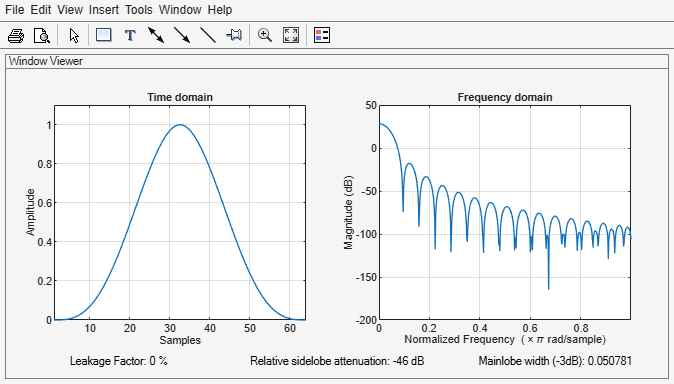Documentation

### This is machine translation

Mouseover text to see original. Click the button below to return to the English version of the page.

Note: This page has been translated by MathWorks. Click here to see
To view all translated materials including this page, select Country from the country navigator on the bottom of this page.

# bohmanwin

## Syntax

``w = bohmanwin(L)``

## Description

example

````w = bohmanwin(L)` returns an `L`-point Bohman window in `w`.```

## Examples

collapse all

Compute a 64-point Bohman window. Display the result using `wvtool`.

```L = 64; bw = bohmanwin(L); wvtool(bw)```## Input Arguments

collapse all

Window length, specified as a positive integer.

Data Types: `single` | `double`

## Output Arguments

collapse all

Bohman window, returned as a column vector.

## Algorithms

A Bohman window is the convolution of two half-duration cosine lobes. In the time domain, it is the product of a triangular window and a single cycle of a cosine with a term added to set the first derivative to zero at the boundary. Bohman windows fall off as 1/w4.The equation for computing the coefficients of a Bohman window is

`$w\left(x\right)=\left(1-|x|\right)\mathrm{cos}\left(\pi |x|\right)+\frac{1}{\pi }\mathrm{sin}\left(\pi |x|\right),\text{ }-1\le x\le 1$`

where x is a length-L vector of linearly spaced values generated using `linspace`. The first and last elements of the Bohman window are forced to be identically zero.

 harris, fredric j. “On the Use of Windows for Harmonic Analysis with the Discrete Fourier Transform.” Proceedings of the IEEE®. Vol. 66, January 1978, pp. 51–83.